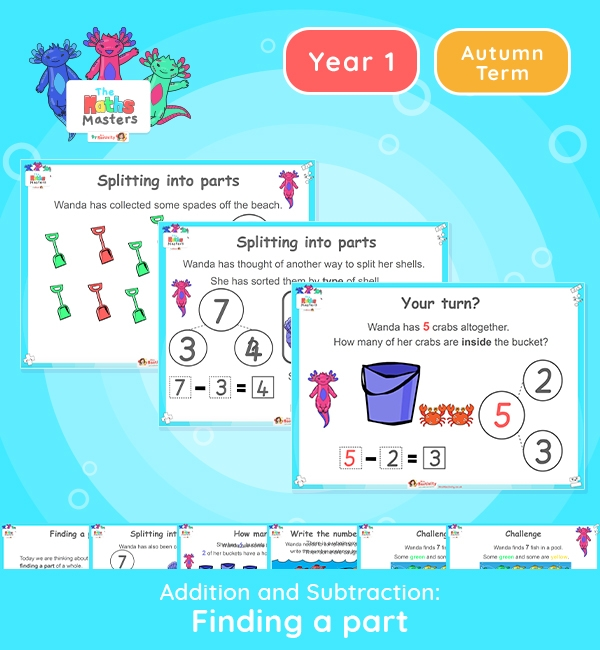# Year 1 | Finding a Part Lesson Presentation## Year 1 addition and subtraction resources

Aligned with the maths mastery approach, this Year 1 | Finding a Part Lesson Presentation is fully editable and designed for the Year 1 maths curriculum to cover the following curriculum objectives:

This lesson focuses on finding one part of a part-whole model by sorting objects into two groups and then recording this as a subtraction sentence.

Small Steps: Subtraction – find a part

NC Links: •Represent and use number bonds and related subtraction facts within 10. •Read, write and interpret mathematical statements involving addition (+), subtraction (-), and equals (=) signs. • Add and subtract one digit numbers to 10, including zero. • Solve one step problems that involve addition and subtraction using concrete objects and pictorial representations.

Ready-to-progress criteria:  1NF-1 Develop fluency in addition and subtraction facts within 10

1AS-2 Read, write and interpret equations continuing addition, subtraction  and equals symbols, and relate additive expressions and equations to real-life contexts.

Previous Experience: Devise and record number stories, using pictures, numbers and symbols.

TAF Statements: Working Towards Add and subtract (one digit numbers) explaining their method verbally in pictures or using apparatus.

Aligned with the order of teaching of the maths mastery approach, use this to help your pupils get to grips with each mathematical concept. This lesson presentation also includes varied fluency activities, problem solving, and mathematical discussion questions too.

Explore our other year 1 addition and subtraction resources.

## Recently Viewed